##Line in trilinear coordinates

Given a triangle ABC, draw the line with given equation Ua*xa + Ub*xb + Uc*xc = Ud in trilinear coordinates. The trilinear coordinates xa, xb, xc denote the oriented distances from the sides of the triangle. Show that the line passes through three points A*, B*, C* on sides BC, CA, AB respectively. Calculate these points in terms of (Ua,Ub,Uc,Ud) and show that: Varying Ui turns the line about I* (i=A,B,C) and varying Ud produces parallel lines.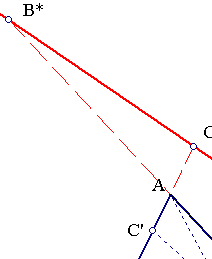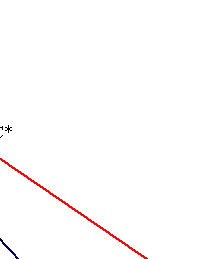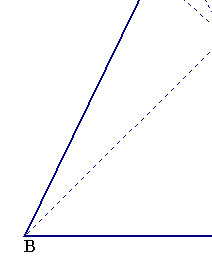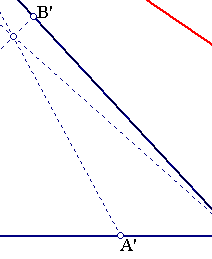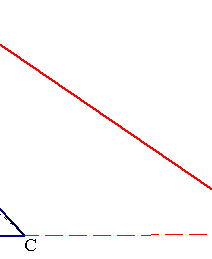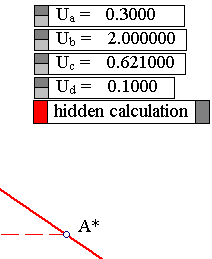A*, B*, C* are the intersection points of the line with the sides of the triangle. They are characterized respectively by xa =0, xb =0, xc = 0. To calculate one of the points, B* say, note that
(1) xb = 0 => [ Ua*xa + Uc*xc = Ud ].
Besides, the point belongs to side AC having equation:
(2) [ a*xa + c*xc - 2D = 0 ], where D the area of the triangle.
Thus the intersection point has coordinates determined by the solution of the linear system:
Ua*xa + Uc*xc = Ud ,
a*xa + c*xc = 2D .
xa = (c*Ud - 2*D*Uc)/(c*Ua - a*Uc), xc = (2*D*Ua -a*Ud)/(c*Ua - a*Uc) ,
(xa/xc) = (c*Ud - 2*D*Uc)/(2*D*Ua -a*Ud).
Provided (Ua : Ub : Uc) is not a multiple of (a, b, c).
Note that equation a*xa + b*xb + c*xc - 2D = 0 is valid for the trilinears of every point of the plane.
The line through B, whose points have distance ratios from the sides BA, BC given by (xa/xc) intersect side AC at the point P=B*, dividing AC in ratio
rb = (B*A/B*C) = -(xc/sinA)/(xa/sinC) = -(xc/xa)*(sinC/sinA) = -(xc/xa)*(c/a) =
= -((2*D*Ua -a*Ud)/(c*Ud - 2*D*Uc))*(c/a) = (ha*Ua - Ud)/(hc*Uc-Ud), where ha is the height to side a.

The intersection point B* of line CA and the line Ua*xa + Ub*xb + Uc*xc = Ud is determined on line CA through the ratio rb. turning property. The other points C*, A* are determined by expressions resulting, from the previous by cyclic substitution of the letters a,b,c. But the equation for rb is independent of Ub This proves the statement about the
Note that there is a double infinity of values of Ui's defining the same line. In fact, the line is uniquely defined by the (oriented) ratios rb = B*A/B*C, rc = C*B/C*A, ra = A*C/A*B, satisfying also the Menelaus condition ra*rb*rc = 1. It follows that for fixed ri's and arbitrary y, and non zero x, all the values of the coefficients given by the following equations determine the same line:
Ud = y,
Ua = (x+y)/ha,
Ub = (x*rc + y)/hb,
Uc = (x*rb*rc + y)/hc.

BarycentricCoordinates.html
BarycentricsFormulas.html
Harmonic.html
TrilinearPolar.html
Trilinears.html
Tripole.html

### References

Yiu, P. Euclidean Geometry Notes (pdf), chapter 8, p. 104. http://www.math.fau.edu/yiu/EuclideanGeometryNotes.pdf